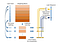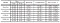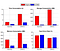# Practical Deep Learning Techniques with Mobile/Edge Computing

Deep learning illustrates outstanding performance in many machine learning tasks, especially in Computer Vision related tasks with large-scale datasets. The prevailing Deep Neural Network (DNN) models are usually highly demanding on computation resources and time. Therefore, traditionally the DL computations are centralized on cloud servers which provide high computing resources.

Edge computing is a distributed computing paradigm that transfers the way of data handling, processing, and delivery closer to users. Edge computing demonstrates the potential to pre-process data and reduce it at local edge/devices before transferring it to the cloud, which is expected to help mitigate the latency, network bandwidth, and data privacy problems mentioned above. However, the challenge is how to address the excessive demand of resources of DL in the edge and device environments.

In this blog post, I will introduce two papers addressing the challenge from different perspectives. The first focuses on DNN model quantization and the second on adopting DL models to edge computing systems.

*The readers are also welcome to read my teammate Charles’s blog about another two papers in this topic.

The first paper is Quantized Convolutional Neural Networks for Mobile Devices by Wu et al in 2016². It focuses on the method to shrink DNN size to speed up test-phase inference time, and the method applies a unified quantization method to both fully connected and convolutional layers.

The paper presents a quantized test-phase computation process of Convolutional Neural Networks (CNN). The quantization method is unified over both the convolutional layers and fully connected layers.The parameter quantization and test-phase computation process of the fully connected layer

Fully Connected Layers:

For the weight matrix W ∈ ℝ^{Cₛ × Cₜ} with Cs rows and Ct columns. The Wcₜ denotes the Ct-th column of the weight matrix. The quantization method consists of the following steps:

1. Evenly split the Cs-dimensional spaces into M subspaces, each of C’ₛ = Cₛ/M dimensions. Each column vector Wcₜ is decomposed into M sub-vectors.
2. Learn a sub-codebook for each subspace using the k-means method. For each subspace, optimize the following equation. The sub-codebook D(m) contains K sub-codewords, and each column in B(m) is an indicator vector (only one non-zero entry).

3 . S(m) is the m-th sub-vector of the layer input. Pre-compute inner products between S(m) and every D(m), store in a look-up table. Then, layer response is approximately computed as:

Convolutional Layers:

For convolutional layers, the method is generally the same. The extra work on the convolutional layer is to first split each convolutional kernel along the dimension of channels. In this way, the pre-computed inner products can be reused at multiple spatial locations. Then, we use the same method with a sliding window in the spatial domain.

The quantization in each subspace is learning to minimize the following equation. pₖ demotes the spatial position at all convolutional kernels.

Then, the layer output is approximated by the following equation, where S^(m)_{pₛ} is the m-th sub-vector at position pₛ in the input feature maps.

Quantization with Error Correction:

To avoid the accumulation of error, the authors further proposed error correction methods to minimize the estimation error.

For the fully connected layers, we optimize the following equation with a block coordinate descent approach. Sn and Tn are layer input and response respectively.

The residual error of the m-th subspace is denoted as

and then we attempt to minimize the residual error of this subspace.

This optimization is solved by alternatively updating the sub-codebook D and sub-codeword assignments B with the two following formulas.

The proposed Quantized-CNN framework can improve the efficiency of the whole CNN models of the following four prevailing models to 4–6× speed-up with minor performance loss of 0.5% — 1.6%, as shown in the table.The speed-up/compression rates and the increase of top 1/5 error rates for the whole CNN model.

The figure illustrates the experiment results of original and quantized AlexNet and CNN-S on a smartphone device. We can see that time, storage, and memory consumption is all significantly reduced while the top-5 error rate has a minor increase.Comparison on the efficiency and classification accuracy between the original and quantized AlexNet and CNN-S on a Huawei R Mate 7 smartphone
• The paper proposed a unified framework to simultaneously accelerate and compress CNNs
• It reaches 4 ∼ 6× speed-up and 15 ∼ 20× compressions with merely one percent loss of classification accuracy in experiments

The second paper is When Deep Learning Meets Edge Computing published in 2017 by Huang et al¹. Despite its concise length, it’s one of the earliest papers to adopt a complete edge computing framework to Deep Learning Models. Therefore, it is a great paper to introduce the readers to Deep Learning models with Edge computing. The paper proposes to deploy models at edge servers to provide timely service to end-users along with pre-processing data to reduce dimension at edge servers.

In the paper, the proposed Edge Learning framework is composed of three main parts:

1. End-user device: data support.
2. Edge server: preliminary processing. The edge servers perform the preliminary process to reduce data dimensionality and reduce data noise.
3. Cluster: execute DNN models.

The end-user devices are responsible for collecting data such as voice and image captures. The data collected is generally large and noisy. Then, at the edge server, we apply data pre-processing methods like Principal Component Analysis (PCA) and Linear Discriminant Analysis (LDA) to the original data. After the preliminary processing, the data dimensionality is reduced and data noise is mitigated. Then, the cleaned and lower-dimensional data is sent to the cluster servers, and the predicted output is returned to end-users.

Furthermore, the model trained on the cloud can be deployed on edge servers to reduce transmission overhead and provide timely services to end-users. The new data can be continuously transferred to the cloud to further update the model.

With 60,000 training data from the MNIST dataset, the learning accuracy can reach 90% and the running time, as well as data transfer traffic, can be significantly reduced.

Figure (a) shows that cooperation with edge computing can significantly reduce the running time despite the size of training data. (b) and (c) illustrates the PCA algorithms can reduce network traffic both between edge and cluster and inter-clusters. Figure (d) shows that with a larger size of data, the accuracy is higher and closer to the accuracies without edge computing. Despite that the PCA would lower the accuracy with small datasets, the accuracy can be improved by increasing the training data size.

• The paper is one of the earliest papers to address the deep learning cloud computing challenge with edge computing solutions.
• It Establishes a framework to distribute a deep learning pipeline between cloud, edge, and devices.

The two papers introduced a focus on compressing CNN models and edge server pre-processing respectively. For the Edge/Cloud partitioning and Device/Edge partitioning, I recommend reading my teammate’s medium blog post link.

Generally, there are two main categories of solutions to accelerate the runtime. Model compression/shrinking, and involvement of computation at edges. The model compression can reduce inference computation time while the involvement of edge computation reduces the transfer latency and mitigates the data privacy issues.

The main concern is the trade-off between accuracy and latency. The papers tackle it by proposing new methods to minimize error and wisely assign the right amount of computation work to edges.

For future work, we notice that none of the paper coordinates the model coordinating on all layers of the device, edge server, and cloud clusters. Also, most of the work focus on VGG and AlexNets, and further exploration can be done on more models like generative models. Moreover, current papers all represent lab experiment results and we are looking to see real-world deployment to verify the performances.

 Huang, Yutao, et al. “When deep learning meets edge computing.” 2017 IEEE 25th international conference on network protocols (ICNP). IEEE, 2017.

 Wu, Jiaxiang, et al. “Quantized convolutional neural networks for mobile devices.” Proceedings of the IEEE Conference on Computer Vision and Pattern Recognition. 2016.

 Canziani, Alfredo, Adam Paszke, and Eugenio Culurciello. “An analysis of deep neural network models for practical applications.” arXiv preprint arXiv:1605.07678 (2016).